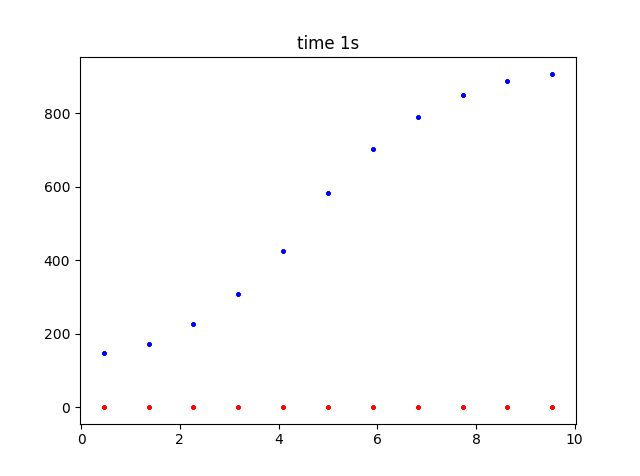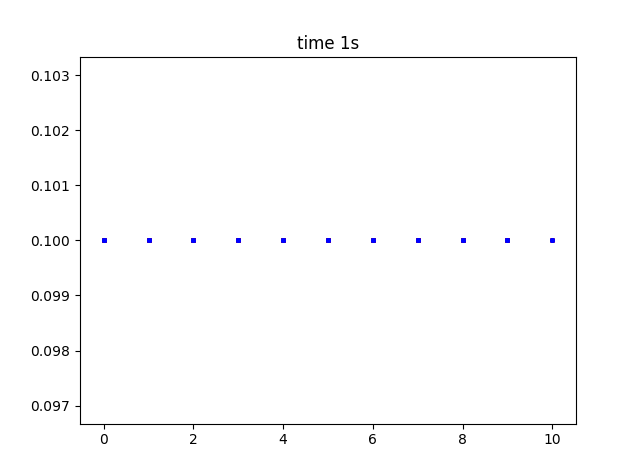Page 1 of 1

### Unexpected 3D behaviour

Posted: Thu Jan 31, 2019 3:24 pm
Sorry. me again. I am confused by the output I get with 3D RXD. Figure I below shows how I get a nice as diffusion if I just add some factor to central nodes with diffusion . It seems to indicate to me I am on the right track.However, now look what happens (2D scatter graphs) when I add a rate reaction.
The first graph here is with solve set to 1D.There is a dear buildup of substance where it is being produced by the rate reaction. (Red is time zero, blue after 1 second).
If I simply change solve type to 3D, I expect to see the same build up of substance in the R.H.S.? see below, I do not.There seem to be no parameters I can use, where species build up when produced by a rate or reaction. I am so sorry, any idea what I an doing wrong? code below:

Code: Select all

``````# -*- coding: utf-8 -*-
"""
Created on Mon Jan 21 19:54:56 2019

@author: Richard
"""

from neuron import h, rxd
from matplotlib import pyplot

# needed for standard run system
h.load_file('stdrun.hoc')

left = h.Section(name='left')

left.nseg= 11
left.L = 10
left.diam= 5

tfact=1.000
rxd.set_solve_type(dimension=3)
#rxd.nthreads(8)
cytosol = rxd.Region(h.allsec(),dx=1)#change dx here.. default 0.25
ctfact = rxd.Species(cytosol, d=.01, initial=0.1)

def right_only(node):
return 1 if node.x3d > left.L/2 else 0

production_region = rxd.Parameter(cytosol, initial=right_only)
reaction = rxd.Rate(ctfact, 1* production_region)
print('about to finalize')
h.finitialize(-60)
print('finalized')

def plot_it(color='b'):
print('plotting')
y = ctfact.nodes.concentration
x = [node.x3d for node in ctfact.nodes]
pyplot.scatter(x, y, marker='o', color=color, s=5)

# plot the initial situation
plot_it('r')

# we will plot every 25ms up to 100ms
h.continuerun(1000)
plot_it()
pyplot.title('time {}s'.format(1))
pyplot.show()``````

### Re: Unexpected 3D behaviour

Posted: Mon Feb 04, 2019 3:05 pm
This is due to a bug in reactions in 3D rxd. Thank you for identifying it.
We have a fix for it, you can clone https://github.com/neuronsimulator/nrn/tree/7.6 and build from source.

Alternatively replace your <neuron python library dir>/neuron/rxd/species.py with https://raw.githubusercontent.com/neuro ... species.py

E.g. on Windows, by default, this file would be
C:\nrn\lib\python\neuron\rxd\species.py
And on mac;
/Applications/NEURON-7.6/nrn/lib/python/neuron/rxd/species.py

### Re: Unexpected 3D behaviour

Posted: Mon Feb 04, 2019 4:19 pm
Oh that's perfect, thanks so much! I did try and debug species.py but of course I got nowhere. Just copying in the new file worked fine-I didn't need to compile.
kind Regards R.